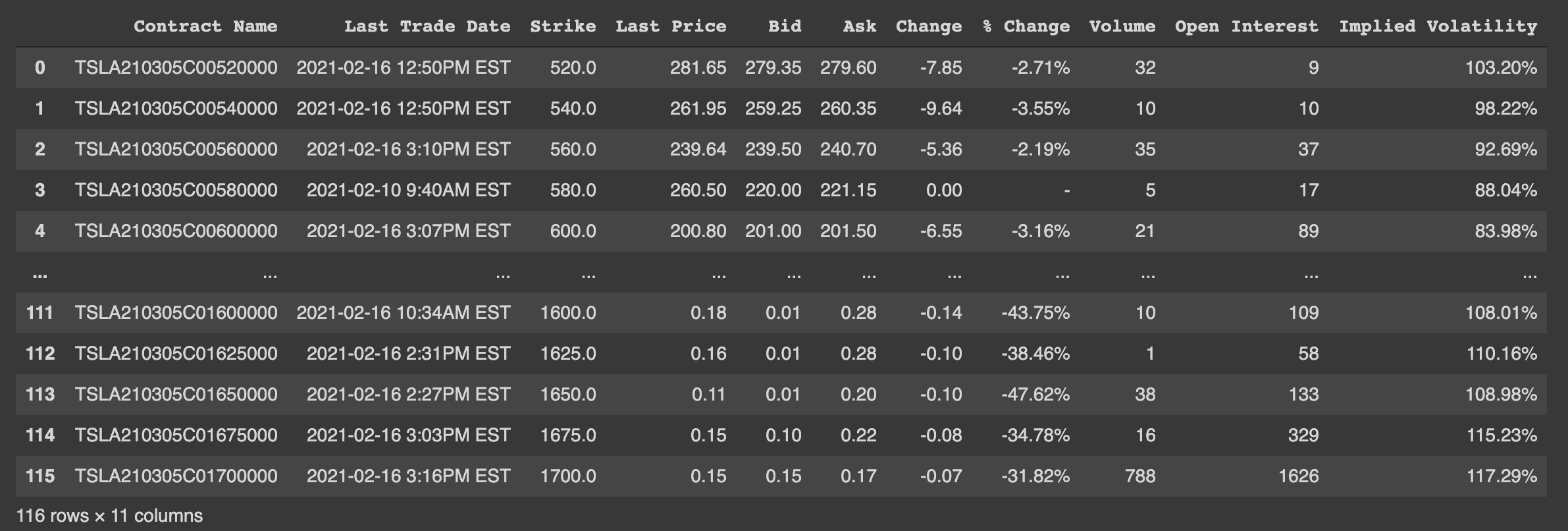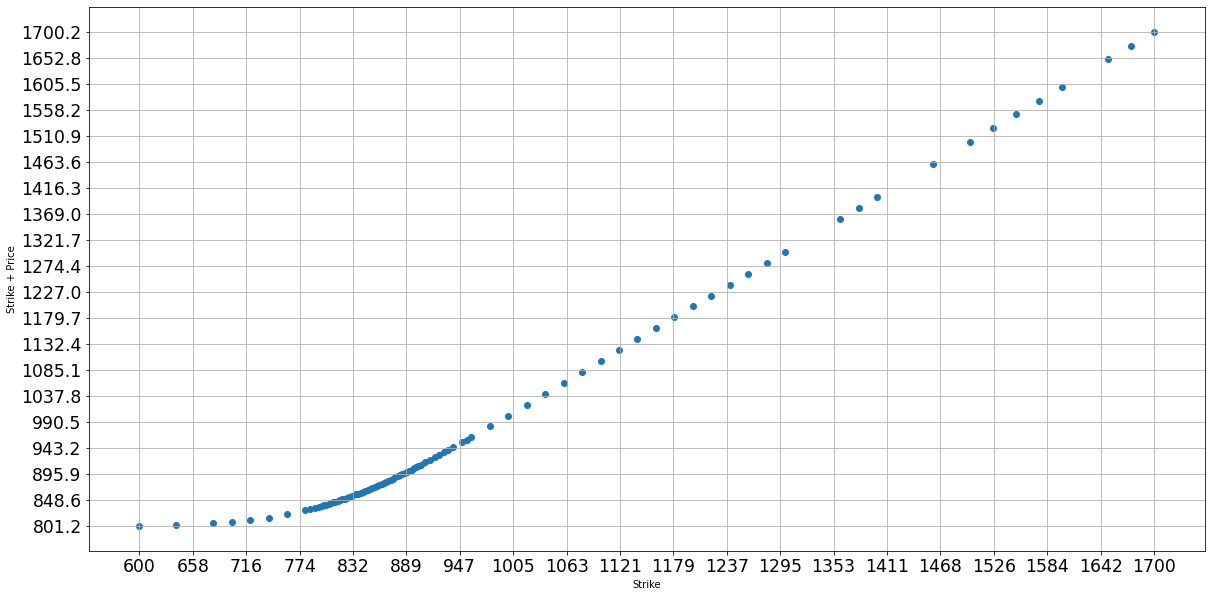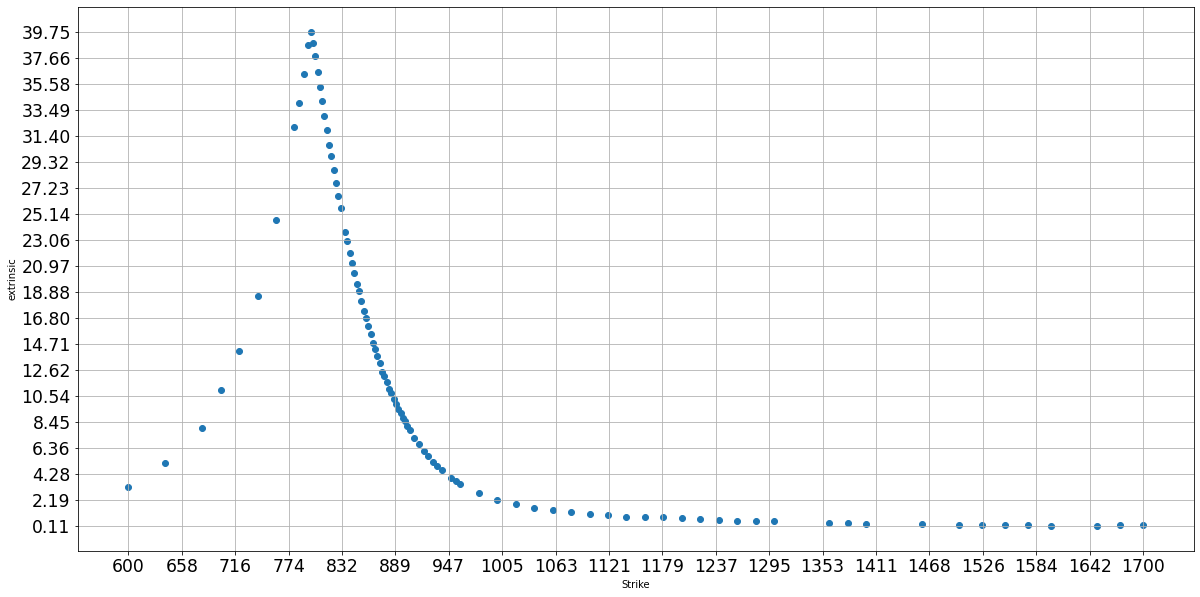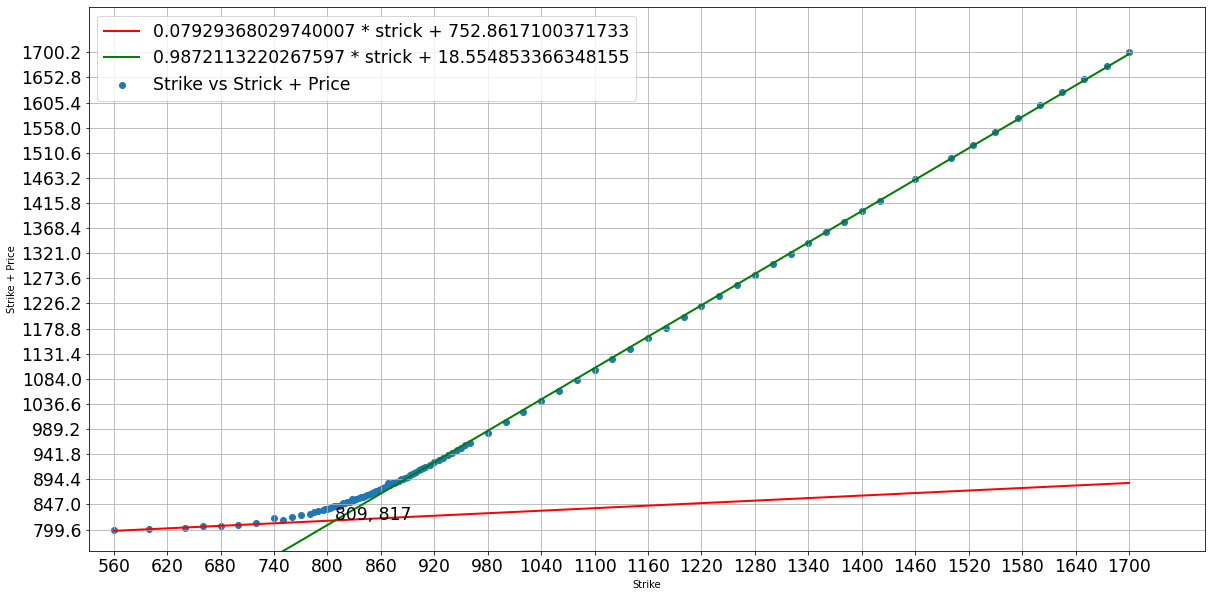# Get Option Data from Yahoo with Pandas

This notebook shows how to read the option chain data from Yahoo finance with Pandas. Especially we will use pandas.read_html.

# Import some Libraries

First, let us import some library

import pandas as pd
from matplotlib import pyplot as plt
import matplotlib
from datetime import datetime
import numpy as np

%matplotlib inline
plt.rcParams['figure.figsize'] = [20, 10]


# Get the Right Url

If we want to get Tesla option data for Mar 5th 2021 from Yahoo finance, we need to visit this url https://finance.yahoo.com/quote/TSLA/options?date=1614902400. TSLA is the ticket name for Tesla and 1614902400 is the unix epoch seconds for Mar 5th 2021. So we have this function

def get_url(tick: str, year: int, month: int, day: int) -> str:
"""Get url for the Yahoo option chain table"""
date = int(datetime(year, month, day).timestamp())
tick = tick.upper()
return f"https://finance.yahoo.com/quote/{tick}/options?date={date}"


# Read Option Data from HTML

Pandas provide a function called read_html which supports import data from web page. This is very convenient if the data you are interested at is well structured in the web page, e.g., a table. This function searches for <table> elements and only for <tr> and <th> rows and <td> elements within each <tr> or <th> element in the table. <td> stands for “table data”. The function signature is:

def read_html(io, match='.+', flavor=None, header=None, index_col=None,
skiprows=None, attrs=None, parse_dates=False,
tupleize_cols=None, thousands=',', encoding=None,
decimal='.', converters=None, na_values=None,
keep_default_na=True, displayed_only=True):


Some important parameters:

• io: A URL, a file-like object, or a raw string containing HTML.
• match: str or compiled regular expression, optional. The set of tables containing text matching this regex or string will be returned. .+ means nonempty string
• flavor: parser of the table. Leave the default one
• header: The row (or list of rows for a MultiIndex) to use to make the columns headers. Leave the default one
• index_col: The column (or list of columns) to use to create the index. Leave the default one
• skiprows: 0-based. Number of rows to skip after parsing the column integer.
• attrs: This is a dictionary of attributes that you can pass to use to identify the table in the HTML. This is important and we will discuss more later.

Note, read_html only supports static page, i.e., javascript will NOT be executed.

attrs allows you specify the rules to match the tables in the HTML. For example, {'id': 'table'} means to find a table whose id is table. For the option table we are interested in, we should search for a table with class puts W(100%) Pos(r) list-options for put options or calls W(100%) Pos(r) Bd(0) Pt(0) list-options for call options.

So this is function we used to fetch option data:

def get_option_data(url: str, call_or_put: bool) -> pd.DataFrame:
"""Get the option data from table

Args:
url: yahoo url
call_or_put: if True, call table will be returned
"""
class_name = "puts W(100%) Pos(r) list-options"
if call_or_put:
class_name = "calls W(100%) Pos(r) Bd(0) Pt(0) list-options"
if len(df) < 1:
raise RuntimeError(f"failed to retrieve data from {url} for {class_name}")
elif len(df) > 1:
print(f"{len(df)} sets of data is found, but 1 is expected")
return df


This is the sample outputs:# Process Data

We want to remove some outliers by filtering out the options which has very low volumne or open interest.

def remove_outlier(df: pd.DataFrame, current_price: float = None):
"""Some price of option are outlier and need to remove"""
# df = df.loc[(df["Change"] > 0.001) | (df["Change"] < -0.001)]
df = df.loc[df["Volume"] != "-"]
df["Volume"] = df["Volume"].astype(int)
df["Open Interest"] = df["Open Interest"].astype(int)
minimum_volume = (df["Volume"] + df["Open Interest"]).quantile(0.1)
return df.loc[(df["Volume"] + df["Open Interest"]) > minimum_volume]


Then let us plot the curve of strike price vs break-even price (strike price + option price):

def plot_strike_profit(df: pd.DataFrame):
"""Plot the strick price vs profit price (strick + cost)"""
strike = df["Strike"]
profit = strike + 0.5 * (df["Bid"] + df["Ask"])
figure = plt.scatter(strike, profit)
plt.ylabel("Strike + Price")
plt.xlabel("Strike")
xticks = np.linspace(np.min(strike), np.max(strike), 20)
yticks = np.linspace(np.min(profit), np.max(profit), 20)
plt.xticks(xticks, fontsize="xx-large")
plt.yticks(yticks, fontsize="xx-large")
plt.grid(True)
return figure


Here we use 0.5 * (df["Bid"] + df["Ask"]) for the option price, as it is more stable than Last Price. This is the plot:Or we could plot strike price vs option extrinsic value:

def plot_strike_extrinsic(df: pd.DataFrame, current_price: float, call_or_put: bool):
"""Plot the strick price vs extrinsic value

Args:
df: option data
current_price: current price of stock
call_or_put: if True, this is call option
"""
strike = df["Strike"]
# extrinsic = price of option if out of money or price of option - abs(strick - current_price)
if call_or_put:
extrinsic = 0.5 * (df["Bid"] + df["Ask"]) - np.maximum(0.0, current_price - df["Strike"])
figure = plt.scatter(strike, extrinsic)
plt.ylabel("extrinsic")
plt.xlabel("Strike")
xticks = np.linspace(np.min(strike), np.max(strike), 20)
yticks = np.linspace(np.min(extrinsic), np.max(extrinsic), 20)
plt.xticks(xticks, fontsize="xx-large")
plt.yticks(yticks, fontsize="xx-large")
plt.grid(True)
return figure


This plot matches the Black Scholes Model described in my previous post.# Some Useless Analysis

I also tried whether I could fit some lines to the curve of strike price vs break-even price.Here is the code.

def fit_line_ransac(x: np.ndarray, y: np.ndarray, error_threshold: float = 1e-3, convergence: float =  0.9, max_iterations: int = 10, initial_sample_size=10):
"""Fit line via ransac"""
number_data = np.prod(x.shape)
sample_size = initial_sample_size
best_quality = 0.0
best_line = None
for i in range(max_iterations):
# random sample the points as initial seed
sampled_indices = np.random.choice(number_data, sample_size)
last_quality = 0.0
while True:
# fit a curve and find how many points lands on this curve
line = np.polyfit(x[sampled_indices], y[sampled_indices], 1)
error = np.abs(y - (line * x + line)) / (np.abs(y) + 1e-3)
sampled_indices = np.where(error <= error_threshold)
fit_quality = np.sum(error <= error_threshold) / number_data
if fit_quality - last_quality <= 1e-3:
# no more new points fitted to this curve
break
if sampled_indices.shape < initial_sample_size:
# too less points lands on the curve
break
# refine the curve with all the points on this curve for robustness
last_quality = fit_quality
# print(f"Fit result at {i} iterations: {fit_quality * 100}% of data points fits to {line}")
if last_quality > best_quality:
best_line = line
best_quality = last_quality
# if most points fit to this curve, then we have found a good curve
# otherwise, try with a new random seed
if fit_quality >= convergence:
break
print(f"Best result: {best_quality * 100}% of data points fits to {best_line}")
return line

def detect_ankle_point(df: pd.DataFrame, current_price: float = None, error_threshold: float = 1e-3):
"""Fit two linear curve and find where it intersects"""
plot_strike_profit(df)
number_data = len(df)
strike = np.array(df["Strike"])
profit = np.array(df["Strike"] + 0.5 * (df["Bid"] + df["Ask"]))

# first curve for bottom 25% of points
if current_price is None:
x = strike[:number_data // 4]
y = profit[:number_data // 4]
else:
x = strike[strike <= current_price * 0.9]
y = profit[strike <= current_price * 0.9]
line1 = fit_line_ransac(x, y, error_threshold=error_threshold)
x = strike[[0, -1]]
y = line1 * x + line1
plt.plot(x, y, color="r", linewidth=2)

# second curve for top 25% of points
if current_price is None:
x = strike[-(number_data // 4):]
y = profit[-(number_data // 4):]
else:
x = strike[strike >= current_price * 1.1]
y = profit[strike >= current_price * 1.1]
line2 = fit_line_ransac(x, y, error_threshold=error_threshold)
x = strike[[0, -1]]
y = line2 * x + line2
plt.plot(x, y, color="g", linewidth=2)

# find the intersection
x = (line2 - line1) / (line1 - line2)
y = line1 * x + line1
plt.annotate(f"{x:.0f}, {y:.0f}", (x, y), fontsize="xx-large")

# change axis
plt.xlim(strike[[0, -1]] * np.asarray([0.95, 1.05]))
plt.ylim(profit[[0, -1]] * np.asarray([0.95, 1.05]))
plt.legend([
f"{line1} * strick + {line1}",
f"{line2} * strick + {line2}",
"Strike vs Strick + Price",
], fontsize="xx-large")
return line1, line2, (x, y)

Written on February 16, 2021